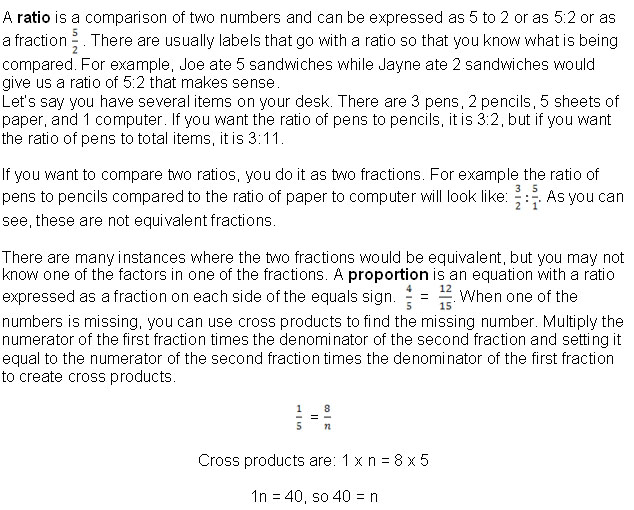Math Skills: Rounding, Exponents, Radicals, Square Roots, and Ratios

 Rounding and Significant Digits

Rounding has to do with significant digits in a number. When working with money, we are limited to dollars and cents. The smallest unit of money in the United States is a penny, so we must have prices that are in whole pennies. Thus when we see a sale that says 30% off and we find an item we want that cost \$24.99 originally, we calculate (0.30) x (24.99) to find out that it is now \$7.497 less than it was. But, wait. We cannot pay \$7.497 less which would be \$17.493 because we cannot pay three tenths of a cent.

We need to round off the number. We can either round off the \$7.497 or the final number of \$17.493. If we round off the percentage off (\$7.497), we look at the digit that indicates cents which is the 9. That is our last significant digit. The next digit, 7, is not significant in that it does not deal with anything we can do. We look to see if it is a 5, 6, 7, 8, or 9. If it is, we round the digit before it up one digit. In this case, the digit is a 9, so by rounding it up one, we actually round the 49 cents to 50 cents. That makes the percentage off \$7.50. Now if we subtract \$7.50 from \$24.99, the new price is \$17.49. If the 7 had been less than 5, we would have simply dropped it, leaving the percentage off as \$7.49 and giving us a new price of \$17.50.

If we wait to round until we have subtracted the percentage off from the original price, we get a new price of \$17.493. The last significant digit is a 9 again, but this time it is followed by a 3, so we drop the 3 because it is less than 5 (0, 1, 2, 3, or 4) and the new price is \$17.49.

Significant figures or significant digits are the digits indicating the precision of a measurement. There is uncertainty only in the last digit.

Let's say you measure an object with a ruler marked in millimeters. The reading on the ruler is found to be about 2/3 of the way between 12 and 13 mm. What value should be recorded for its length? Recording 13 mm does not give all the information that you found. Recording 12 2/3 mm implies that an exact ratio was determined. Recording 12.666 mm gives more information than you found. A value of 12.7 mm or 12.6 mm should be recorded because there is uncertainty only in the last digit.

Determining the number of significant digits in a quantity:

1) All nonzero digits are significant and all zeros between nonzero digits are significant.

4.521 and 7002 both have four significant digits.

2) Zeros to the left of the first nonzero digit are not significant.

0.0002 contains one significant digit.

3) Zeros to the right of a non-zero digit and the decimal point are significant figures.

32.500 g contains five significant digits.

4) The significance of numbers ending in zeros that are not to the right of the decimal point can be unclear, so this situation should be avoided by using scientific notation (explained later) or a different decimal prefix. Sometimes a decimal point is used as a placeholder to indicate the units-digit is significant. A word like "thousand" or "million" may be used in informal contexts to indicate the remaining digits are not significant.

"1200 nails" would be considered to have four significant digits,

but in the sentence, "We will need 1100 to 1200 nails for the roof,"

it almost certainly has only two.

5) Exact numbers have no uncertainty and contain an infinite number of significant digits. These relationships are definitions. They are not measurements.

There are exactly 1000 L in one cubic meter.

Rules for rounding off significant digits:

1)  If the leftmost digit to be removed is a four or less, then round down. The last remaining digit stays as it was.

Rounding 43.4 to two significant digits gives 43.

2) If the leftmost digit to be removed is a five or more, then round up. The last remaining digit increases by one.

Rounding 6.77 to two significant digits gives 6.8.

Rules for calculating with significant figures:

1) For multiplication or division, the result has the same number of significant digits as the term with the least number of significant digits.

2.54 x 3.1 = 7.874

The term with the least number of significant digits is 3.1 with 2 significant digits.

Interested in learning more? Why not take an online Basic Math course?

Therefore, you must round to 2 significant digits: 7.9

Note that this is not digits after the decimal point;

it is total significant digits.

2)  For addition or subtraction, the result has the same number of digits after the decimal point as the term with the least number of digits after the decimal point.

250.0 +  26 + 4.73

Add the three numbers as they are: 280.73

One of these numbers has no digits after the decimal point,

so our final number will have no digits after the decimal point: 281.

3)  For multi-step calculations, maintain all significant digits when using a calculator or computer and round off the final value to the appropriate number of significant digits after the calculation. When calculating by hand or when writing down an intermediate value in a multi-step calculation, maintain the first non-significant digit.

 Estimating

Estimating takes rounding to another level. If you want to add two numbers by estimating, you will first round them. Let's say you want to add 654 and 35. It would be easy to estimate 654 as 650 and then add 35 to it to get 685. However, if you want to add 654 + 35 + 567 + 82, it becomes a bit more difficult. You could estimate them as 650 + 40 + 570 + 80 and get 1340 or you could round 654 to 700, 35 to 30 since you estimated the 654 high, 567 as 600, and 82 as 80 which would give you 700 + 30 + 600 + 80 = 1410. The real answer to 654 + 35 + 567 + 82 is 1338, so your first estimate is quite close.

Subtracting usually is a little trickier in that sometimes the numbers are pretty close together. It works well if you have 625 – 56 because you can figure out 50 from 625. However, if you want to subtract 47.35 from 50.54, you cannot estimate both numbers at 50! You need to see that the decimal portion of both numbers is about half or less and figure the difference between 47.5 and 50.5 to be about 3 and the difference between 0.35 and 0.54 is about 0.2, so your estimate would be 3.2. The real difference is 3.19.

Becoming proficient at estimating takes a lot of practice. However, it is good to be able to estimate answers. That is an easy way to figure out possible answers on a quiz or test or to decide if you are doing a problem correctly. Often by estimating, you can realize that two answers out of four in a multiple choice problem are not possible. By estimating, you can quickly decide how many cans of paint to buy or if you can afford a car payment of a particular amount.

 Powers and Exponents

There is a simple way to show that a number is multiplied by itself. If you want to multiply 2 x 2, you could show it as 22 which is called "two squared" or "two to the second power." If you want to multiply 2 x 2 x 2, it is 23 which is "two cubed" or "two to the third power. It is helpful to memorize squares and cubes of the numbers 1 through 15 as they are used often.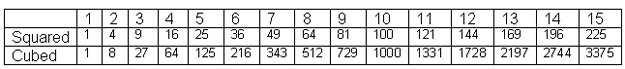There are times when these numbers will be taken to other powers. In each case, look at the exponent (the number that indicates the power and is located as a superscript) and multiply the number times itself that many times. For example, 85 = 8 x 8 x 8 x 8 x 8 = 32,768.

One place that exponents are used is scientific notation (explained later in this chapter). In this case the exponent is always with 10 because it denotes place value. 100 is equal to 1. (In fact, any number to the zero power is one.) 101 = 10, 102 = 100, 103 = 1,000 and so forth.

5.2358. x 103

This really means 5.2358 x 10 x 10 x 10

Or 5.2358 x 1,000

Count to the right 3 places to find out what the number is

when it is not in scientific notation: 5235.8

We can also have negative exponents. When 10 has a negative exponent, it denotes a decimal place. 10-1 = 0.1 while 10-4 = 0.0001.

4.863 x 10-3

This really means 4.863 x 10-1 x 10-1 x 10-1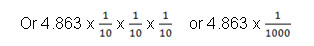Count to the left three places to find out what the number is

When it is not in scientific notation: 0.004863

Some things to remember about exponents:

1) Zero to any power is still zero (04 = 0)

2) One to any positive power is one (15 = 1)

3) Any number to the zero power is one (50 = 1, 100 = 1)

4) Any number to the first power is that number (41 = 4, 91 = 9)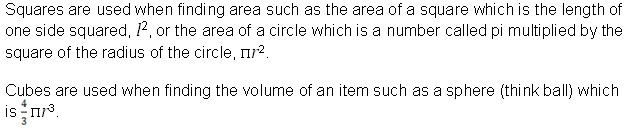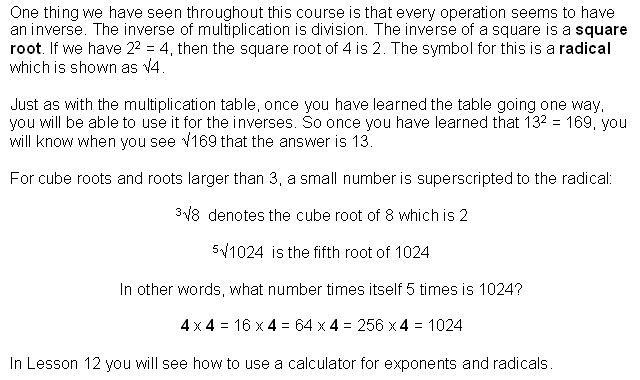Scientific Notation and Significant Digits

In science extremely large and extremely small numbers are often encountered. A simpler method of writing these numbers and denoting significant figures is called scientific notation. In scientific notation, a number is written in the standard form of M x 10n where M is a single digit number from 1 to 9 and the exponent n is a positive or negative integer. If the decimal point was moved to the right to create a number from 1 to 9, then n is negative.

0.00000356 would become 3.56 x 10-6

If the decimal point was moved to the left to create a number from 1 to 9, then n is positive.

144,267,000 would be 1.44267 x 108.

Working with numbers in Scientific Notation:

·       To add or subtract numbers in scientific notation, the exponent n must be the same for all the numbers involved.

To add 1.39 x 106 and 5.27 x 108

you could change 5.27 x 108 to 527 x 106

so the end result would be 528.39 x 106

or you could change 1.39 x 106 to 0.0139 x 108

and add it to 5.27 x 108 to get 5.2839 x 108

which would keep the result in correct scientific notation.

Each of the original numbers had three significant figures,

so the answer has three significant figures: 5.28 x 108.

·       To multiply numbers in scientific notation, multiply the numbers (the M part) and then add the exponents.

Multiply 5.2 x 105 by 6.8 x 102

Multiply 5.2 x 6.8 = 35.36

and then add the exponents to get 105 + 2 = 7.

Now you have 35.36 x 107 which is not in correct scientific notation.

To put it into correct scientific notation,

move the decimal point to get 3.536 x 108.

However, each of the numbers multiplied had only two significant figures,

so the answer will only have two, giving you 3.5 x 108.

·       To divide numbers in scientific notation, divide the numbers (the M part) and then subtract the exponents and move the decimal point as needed.

Divide 5.2 x 105 by 6.8 x 102

Start by dividing 5.2 by 6.8 such that 5.2/6.8 = 0.7647

and then subtract exponents to get 105 – 2 = 3.

You now have 0.7647 x 103.

Move the decimal point to get 7.647 x 102.

To get the correct number of significant figures,

look at the original numbers which each had two

and round the answer accordingly: 7.6 x 102.

·       To square a number in scientific notation, square the M value and double the exponent.

(1.2 x 103)2 = 1.4 x 106.

·       To find the square root, the exponent needs to be an even number. If it is an odd number, move the decimal one place to make M a number from 10 to 99. Then determine the square root of M and divide the exponent, n, by 2.

The square root of 2.5 x 107

will be determined as the square root of

25 x 106 which is 5 x 103.

 Ratios and Proportions